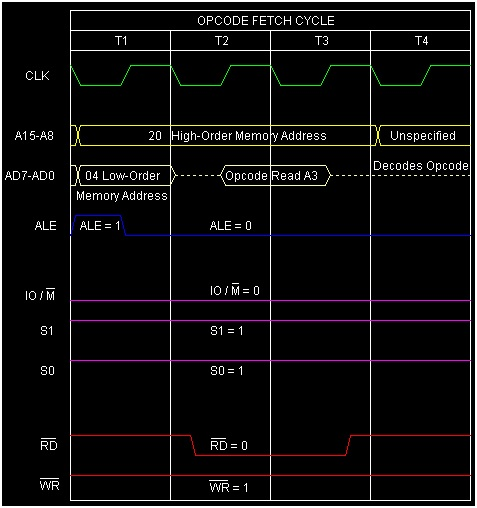# Instruction type ANA R in 8085 Microprocessor

In 8085 Instruction set, ANA is a mnemonic, which stands for “ANd Accumulator” and “R” stands for any one of the following registers, or memory location M pointed by HL pair.

R = A, B, C, D, E, H, L, or M


This instruction is used to AND contents of R with Accumulator’s content. The result thus produced for this AND operation will be stored back in to the Accumulator over writing its previous content. As R can have any of the eight values, there are eight opcodes for this type of instruction. It occupies only 1-Byte in memory.

Mnemonics, Operand Opcode(in HEX) Bytes
ANA A A7 1
ANA B A0 1
ANA C A1 1
ANA D A2 1
ANA E A3 1
ANA H A4 1
ANA L A5 1
ANA M A6 1

Let us consider ANA E is an example instruction of this type. As it is a 1-Byte instruction so in memory only a single Byte will get allocated for it during execution. Let us consider the initial content of Accumulator is ABH and register E is CDH. So after The result of execution of this instruction has been depicted in the following tracing table −

Before After

(E)

CDH CDH

(A)

ABH 89H

(F)

Any values Cy=0,AC=1,Z=0,P=0,S=1

The internal calculation will be done as shown below −

(A) ABH ---> 1010 1011
(d8) CDH ---> 1100 1101
---------
ANA E ---> 1000 1001 (89H)